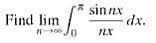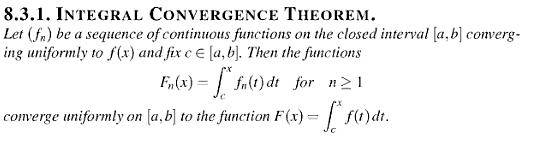# Find the Limit of the Integral

## Homework Statementhint: find the limit of the integral over [ε,pi] and estimate the rest.

## The Attempt at a SolutionI think it is related to the integral convergence theorem, but not all conditions are satisfied. To use the theorem, maybe we have to break the integral into two parts and integrate over [ε,pi]? But how?

But I'm still not sure how we can solve this.

Any help would be much appreciated!

Last edited:

Hint: Don't use that theorem and attack the problem in a different way.

That would be weird as this problem is coming out directly from the section about the integral convergence theorem in my textbook, so they should be related...

If we instead break the integral into two parts [0,ε], [ε,pi], can we use the integral convergence theorem to interchange the lim and integral signs and evaluate?

That would be weird as this problem is coming out directly from the section about the integral convergence theorem in my textbook, so they should be related...

If we instead break the integral into two parts [0,ε], [ε,pi], can we use the integral convergence theorem to interchange the lim and integral signs and evaluate?

I think you have the right idea. As for the interval $[0,\epsilon]$, consider using

$$\left| \int_I f \right| \le \int_I |f|$$

I think you have the right idea. As for the interval $[0,\epsilon]$, consider using

$$\left| \int_I f \right| \le \int_I |f|$$

Sorry, I don't get your hint, why are we doing this?

Also, how can we prove that all conditions in the integral convergence theorem are satisfied? How can we show unifromly convergence of the seqeuence of functions, as required by the theorem?

Thanks!

To do it in the requested way requires that you already know the answer (that's why I don't like this approach).

The integral from delta to pi can be treated using the theorem as you have unifoirm convergence on that interval. The integral from zero to delta can be shown to be of order delta (rs1n's argument will lead you to that).

Now that uniform convergence is to a function identical to zero, so all this amounts to the following. Given any epsilon > 0, you can find a delta which proves that the absolute value of the integral is less than epsilon. Therefore the integral has to be zero.

Let fn(x)=sin(nx)/nx.

But fn(x) converges uniformly to WHAT function? How can we systematically figure out this limit function? Also, is the limit function the same for ALL values of x?

Thanks!

Last edited:
Let fn(x)=sin(nx)/nx.

But fn(x) converges uniformly to WHAT function? How can we systematically figure out this limit function? Also, is the limit function the same for ALL values of x?

Thanks!

|sin(nx)| <= 1

n x tends to infinity for x > 0 and n to infinity

|sin(nx)| <= 1

n x tends to infinity for x > 0 and n to infinity

Why does this imply fn(x)=sin(nx)/nx is uniformly convergent?

To show uniform convergence, we need to prove that:
sup|fn(x)-0| ->0 as n->infinity
ε≤x≤pi

But what is
sup|fn(x)-0| ??? How can we evaluate this supremum?
ε≤x≤pi

Thanks!

Last edited:
Why does this imply fn(x)=sin(nx)/nx is uniformly convergent?

To show uniform convergence, we need to prove that:
sup|fn(x)-0| ->0 as n->infinity
ε≤x≤pi

But what is
sup|fn(x)-0| ??? How can we evaluate this?
ε≤x≤pi

Thanks!

$$\left|\frac{\sin(nx)}{nx}-0\right| = \frac{|\sin(nx)|}{nx} = \frac{1}{n}\cdot \frac{|\sin(nx)|}{x}$$

What is the largest value of $\frac{|\sin(nx)|}{x}$? The most it can be is the maximum of the numerator divided by the minimum of the denominator. Can you take it from there? (Remember that $x\in[\epsilon, \pi]$)

As for my earlier hint, compare sin(t) vs t if |t|<1.

$$\left|\frac{\sin(nx)}{nx}-0\right| = \frac{|\sin(nx)|}{nx} = \frac{1}{n}\cdot \frac{|\sin(nx)|}{x}$$

What is the largest value of $\frac{|\sin(nx)|}{x}$? The most it can be is the maximum of the numerator divided by the minimum of the denominator. Can you take it from there? (Remember that $x\in[\epsilon, \pi]$)

As for my earlier hint, compare sin(t) vs t if |t|<1.

hmm...I sitll don't get it.

I think the largest value is attained at x = pi/(2n), but then
sup|fn(x)-0| is NOT going to converge to 0.
ε≤x≤pi

Also, I think pi/(2n) has some problems too...if n is large enough, it will be less than ε which is nonsense.

So what is the answer of
sup|fn(x)-0| ??
ε≤x≤pi

hmm...I sitll don't get it.

I think the largest value is attained at x = pi/(2n), but then
sup|fn(x)-0| is NOT going to converge to 0.
ε≤x≤pi

Also, I think pi/(2n) has some problems too...if n is large enough, it will be less than ε which is nonsense.

So what is the answer of
sup|fn(x)-0| ??
ε≤x≤pi

When proving that $f_n(x) \to f(x)$ uniformly, you simply need to show that given $\epsilon>0$ you can find an integer N (that is independent of x) such that for all $n>N$, we have $|f_n(x) - f(x)| < \epsilon$ for all relevant x. Even if you do consider the values $\frac{\pi}{2n}$ and substitute them into the fraction $\frac{|\sin(nx)|}{nx}$, the results are all still less than $\frac{1}{n\cdot \epsilon}$.

OK!

But still how can we eavluate
sup|fn(x)-0| ?
ε≤x≤pi

The largest value the numerator can take is 1, and the smallest value the denominator can take is nε, but they do not necessarily correspond to the same point, i.e. sin(nε) is not necessarily equal to 1.

In other words, it is not necessarily true that
sup|fn(x)-0| =1/(nε)
ε≤x≤pi

The largest value the numerator can take is 1, and the smallest value the denominator can take is nε, but they do not necessarily correspond to the same point, i.e. sin(nε) is not necessarily equal to 1.

Why does that matter? Consider the list A of all the possible values of $\sin(nx)$ for $x\in[\epsilon,\pi]$. Then consider the list B of all the possible values of $nx$ for $x\in[\epsilon,\pi]$. What is the largest possible fraction you can make if your numerator must come from the list A, and your denominator is from the list B. The most the fraction can ever be will consist of the largest number from A as the numerator, and the smallest number from B as the denominator. What does it matter that the numerator was obtained using a different value of x than that of the denominator?

How do you compare two fractions a/b and c/d? If b=d, then we know the larger fraction is the one with the larger numerator. If a=c, then we know that the larger fraction is the one with the smaller denominator. And in general, the larger fraction will have a larger numerator and smaller denominator.

Just think for a moment. Let's say you found a magical value $x_0\in [\epsilon,\pi]$ such that
$$\frac{|\sin(nx_0)|}{nx_0}$$
the absolute maximum. Well, is it possible that the numerator will ever exceed 1 for this particular $x_0$? No. And is it true that $nx_0\ge n\epsilon$? YES. Therefore even this supposed absolute maximum is at most $\frac{1}{n\epsilon}$.

Last edited:
$$\frac{|\sin(nx)|}{nx} \le \frac{1}{nx}$$

and

$$\frac{1}{nx} \le \frac{1}{n\cdot \epsilon}$$

Put the two together.

My point is that we cannot say the sup is EQUAL to 1/(nε)
sup|fn(x)-0| = 1/(nε)
ε≤x≤pi

We can only say that the sup is less than or equal to 1/(nε)
sup|fn(x)-0| ≤ 1/(nε)
ε≤x≤pi

But I guess that doesn't matter. If ε is fixed, then 1/(nε) ->0 as n->infinity, and so by the squeeze theorem, the sup goes to 0 as well. Thus fn is unifomly convergent to 0 on [ε, pi].

Let fn(x)=sin(nx)/nx.

Let ε be a fixed number between 0 and pi.

Since fn is continuous on [ε,pi] and fn converges unfiromly to 0 on [ε,pi], by the integral convergence theorem,

pi
∫ sin(nx)/(nx) dx ->0 as n->infinity
ε

But now how can we find the limit of
ε
∫ sin(nx)/(nx) dx as n->infinity?
0

Thanks!

My point is that we cannot say the sup is EQUAL to 1/(nε)
sup|fn(x)-0| = 1/(nε)
ε≤x≤pi

We can only say that the sup is less than or equal to 1/(nε)
sup|fn(x)-0| ≤ 1/(nε)
ε≤x≤pi

But I guess that doesn't matter. If ε is fixed, then 1/(nε) ->0 as n->infinity, and so by the squeeze theorem, the sup goes to 0 as well. Thus fn is unifomly convergent to 0 on [ε, pi].

Correct; you do not need it to be equal because in your definition of uniform convergence you must then take the limit of the sups. That is, $f_n \to f$ uniformly on E if

$$\lim_{n\to\infty} \sup_{x\in E} |f_n(x)-f(x)|=0$$

(I presume you knew this already).

But now how can we find the limit of
ε
∫ sin(nx)/(nx) dx as n->infinity?
0

Thanks!

Here, you approximate the maximum value of the integral. For example, if $|f(x)| \le M$ on the interval $I=[a,b]$, then

$$\left| \int_I f \right| \le \int_I |f| \le \int_I M = (b-a)\cdot M$$

which can be as small as we want if we choose the interval appropriately.

Here, you approximate the maximum value of the integral. For example, if $|f(x)| \le M$ on the interval $I=[a,b]$, then

$$\left| \int_I f \right| \le \int_I |f| \le \int_I M = (b-a)\cdot M$$

which can be as small as we want if we choose the interval appropriately.
OK, so in our case,
|sin(nx)/(nx)|≤ 1/(n|x|) ≤ ???
What is the upper bound on the right side? What if x is very small??

OK, so in our case,
|sin(nx)/(nx)|≤ 1/(n|x|) ≤ ???
What is the upper bound on the right side? What if x is very small??

Compare sin(t) vs t for |t|<1.

Compare sin(t) vs t for |t|<1.

Using a graphing calculator, it seems like sin(t)≤|t|, but how can we obtain and prove this by hand?

Using a graphing calculator, it seems like sin(t)≤|t|, but how can we obtain and prove this by hand?

Look at $f(t)=\sin(t)-t$ as a function. What can you say about the derivative for 0<|t|<1. And since f(0)=0, then the graph of f(t) must be where, relative to the x axis?

So we showed that
lim ∫ sin(nx)/(nx) dx ≤ ε + 0 = ε
n->oo
where ε is some FIXED number between 0 and pi.
note: ∫ is from 0 to pi

But this only gives an upper bound on our answer. How can we find the exact value of lim ∫ sin(nx)/(nx) dx?

Thanks!

The statement is valid for any arbitrary epsilon > 0. So, the absolute value of the integral is less than any arbitrary positive number.